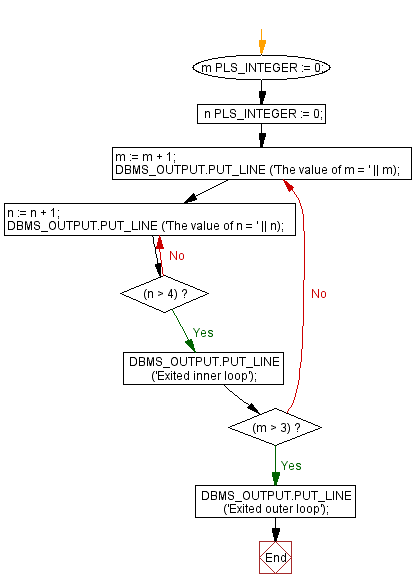﻿ PL/SQL Control Statement: Using nested loop with EXIT WHEN statement - w3resource# PL/SQL Control Statement Exercises: Using nested loop with EXIT WHEN statement

## PL/SQL Control Statement: Exercise-20 with Solution

Write a program in PL/SQL using nested loop with EXIT WHEN statement.

Sample Solution:

PL/SQL Code:

``````DECLARE
m PLS_INTEGER := 0;
n PLS_INTEGER := 0;

BEGIN
LOOP
m := m + 1;
DBMS_OUTPUT.PUT_LINE ('The value of m = ' || m);

LOOP
n := n + 1;
DBMS_OUTPUT.PUT_LINE ('The value of n = ' || n);
EXIT WHEN (n > 4);
END LOOP;

DBMS_OUTPUT.PUT_LINE ('Exited inner loop');

EXIT WHEN (m > 3);
END LOOP;

DBMS_OUTPUT.PUT_LINE ('Exited outer loop');
END;
/
```
```

Flowchart:Sample Output:

```The value of m = 1
The value of n = 1
The value of n = 2
The value of n = 3
The value of n = 4
The value of n = 5
Exited inner loop
The value of m = 2
The value of n = 6
Exited inner loop
The value of m = 3
The value of n = 7
Exited inner loop
The value of m = 4
The value of n = 8
Exited inner loop
Exited outer loop

PL/SQL procedure successfully completed.```

Improve this sample solution and post your code through Disqus

What is the difficulty level of this exercise?

﻿